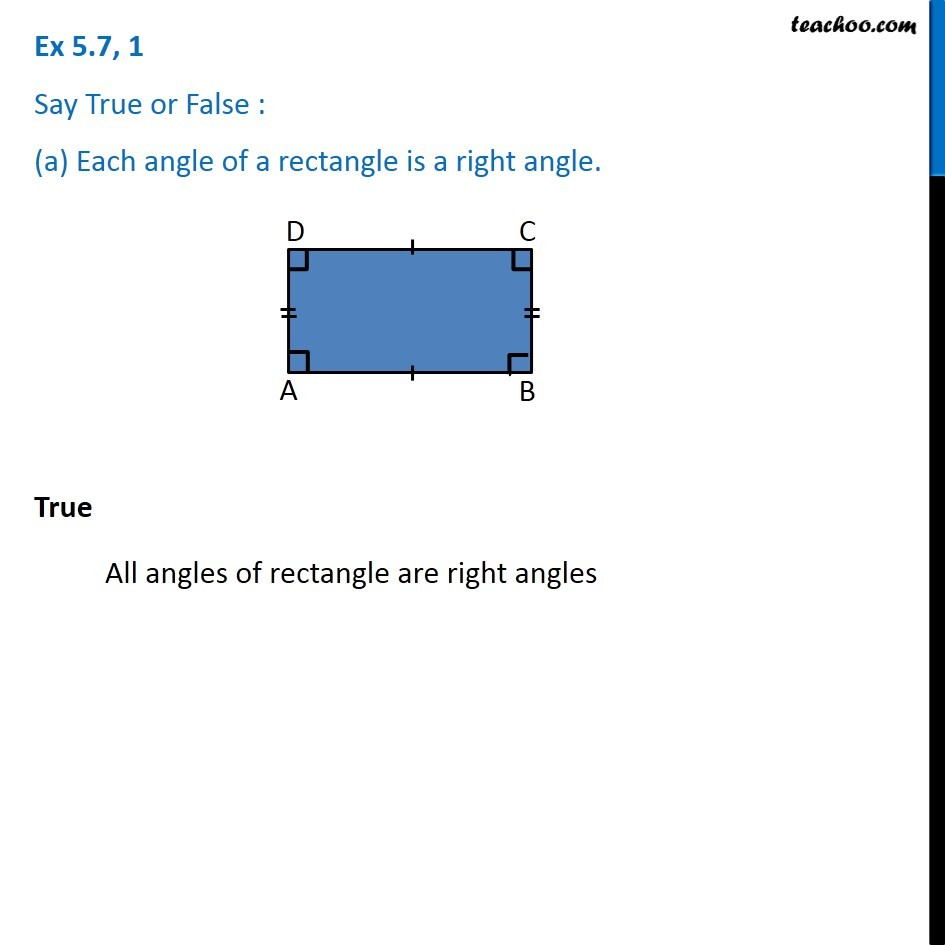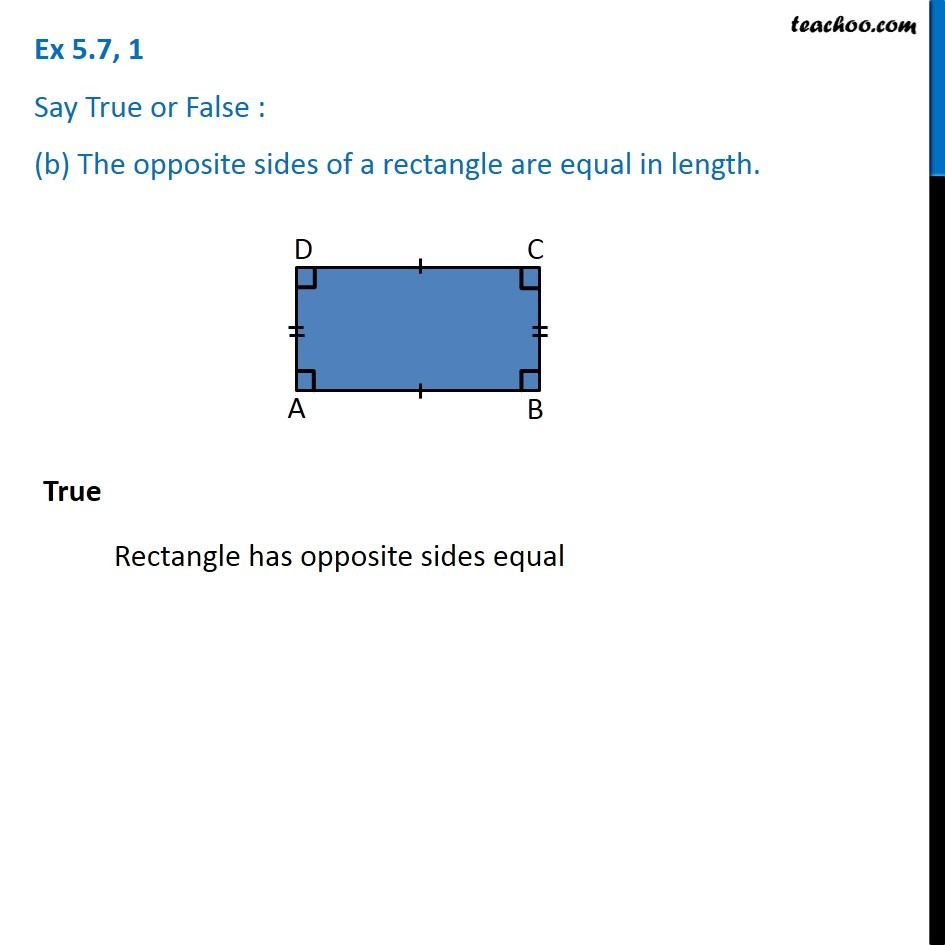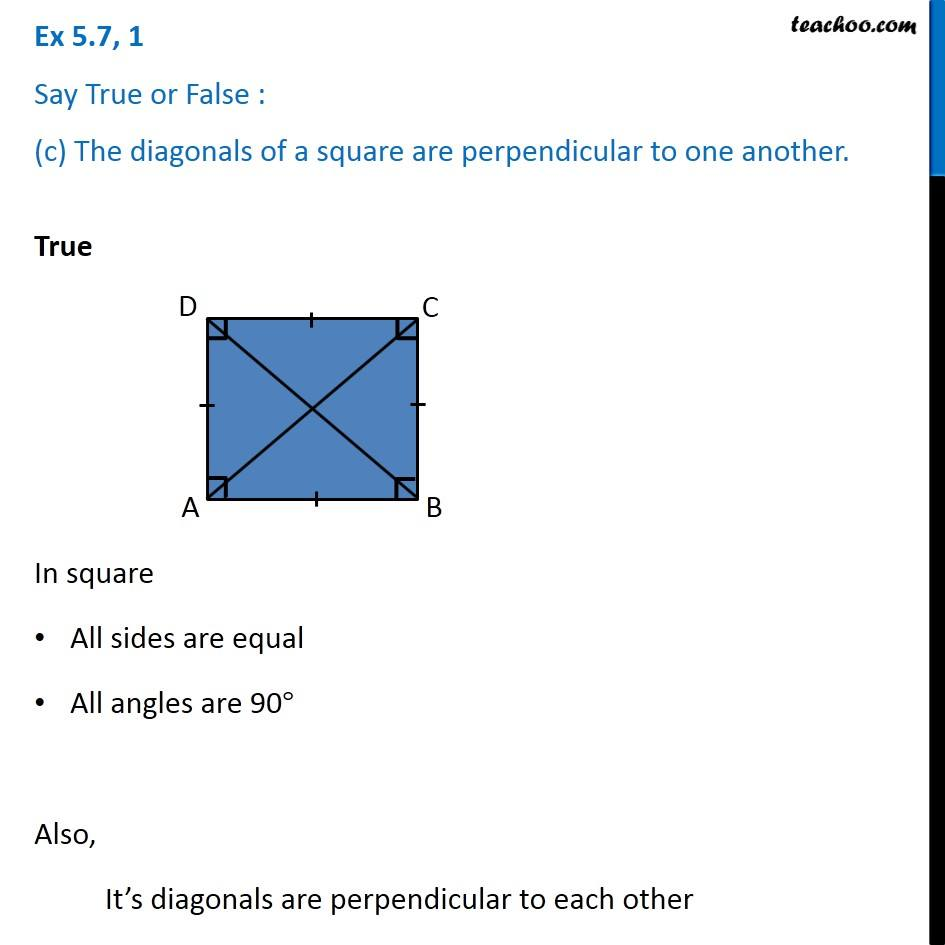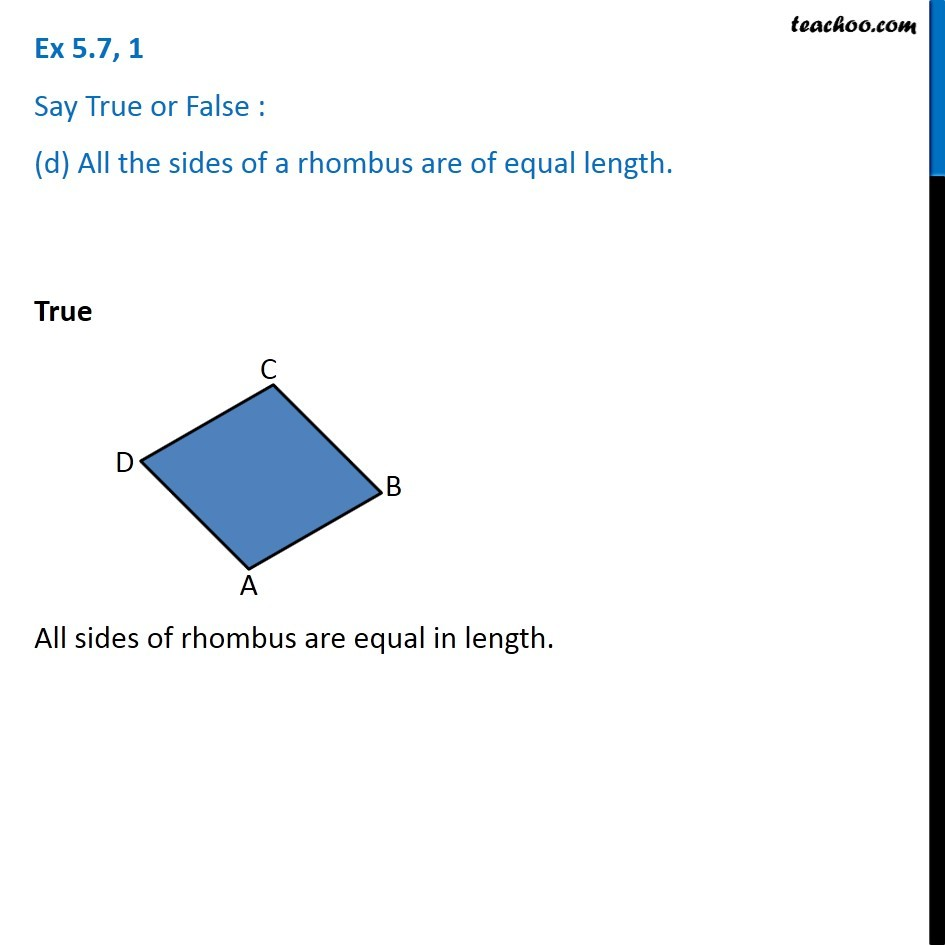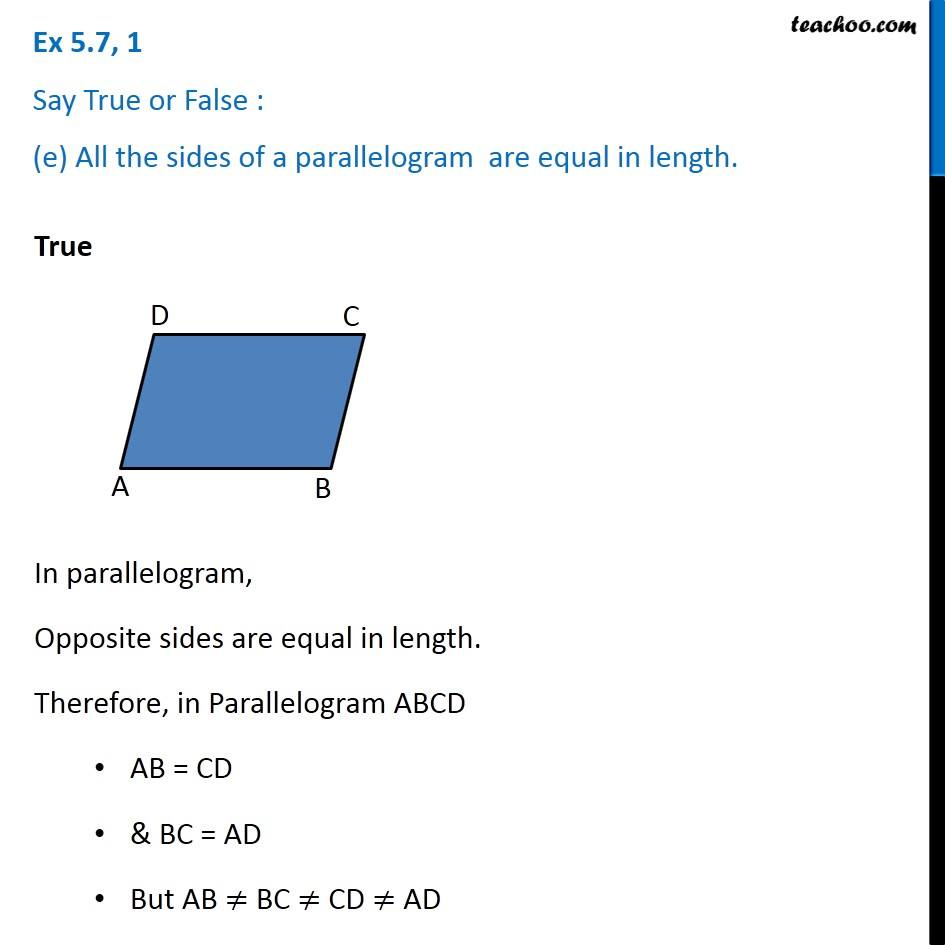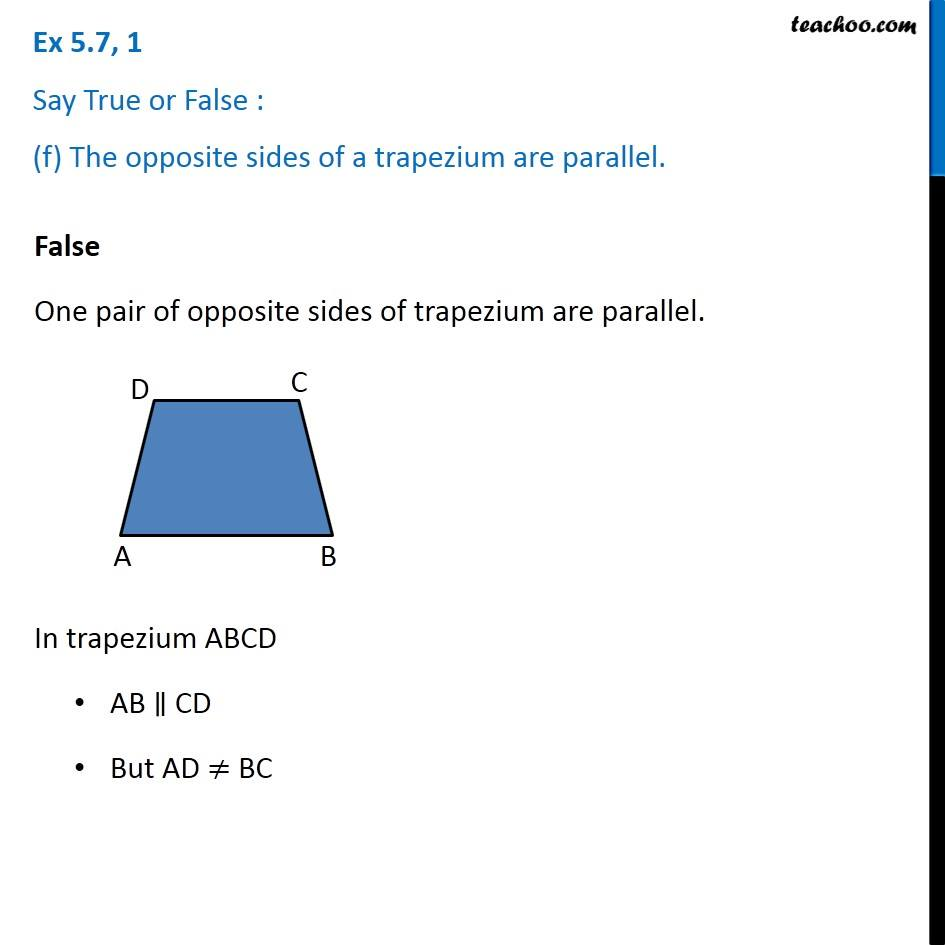Subscribe to our Youtube Channel - https://you.tube/teachoo

1. Chapter 5 Class 6 Understanding Elementary Shapes
2. Serial order wise
3. Ex 5.7

Transcript

Ex 5.7, 1 Say True or False : (a) Each angle of a rectangle is a right angle. True All angles of rectangle are right angles Ex 5.7, 1 Say True or False : (b) The opposite sides of a rectangle are equal in length. True Rectangle has opposite sides equal Ex 5.7, 1 Say True or False : (c) The diagonals of a square are perpendicular to one another. True In square All sides are equal All angles are 90° Also, It’s diagonals are perpendicular to each other Ex 5.7, 1 Say True or False : (d) All the sides of a rhombus are of equal length. True All sides of rhombus are equal in length. Ex 5.7, 1 Say True or False : (e) All the sides of a parallelogram are equal in length. True In parallelogram, Opposite sides are equal in length. Therefore, in Parallelogram ABCD AB = CD & BC = AD But AB ≠ BC ≠ CD ≠ AD Ex 5.7, 1 Say True or False : (f) The opposite sides of a trapezium are parallel. False One pair of opposite sides of trapezium are parallel. In trapezium ABCD AB ∥ CD But AD ≠ BC

Ex 5.7